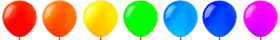# Free Online Play: learn the Multiplication Table

These play practice will help teach your child to count multiplication examples quickly and correctly. Problem has different levels of complexity, consider the pace of learning of the child, the level of formation of its computing skills.

In order for a child to improve multiplication skills, it is necessary to practice every day. Gradually move to a new level of difficulty. Acquired skills must be used in everyday life. Tasks will promote the development of attention, memory, oral and written multiplication skills.

Lesson
2
3
4
5
6
7
8
9### Multiplication tables up to 9

Practice each timetable separately until you master it

Multiply by
2
Multiply by
3
Multiply by
4
Multiply by
5
Multiply by
6
Multiply by
7
Multiply by
8
Multiply by
9### Practice a mix of multiplication facts

Multiplication table
true or false
Multiplication table
which is true?
Multiplication table
select missing numbers
Multiplication table
Test